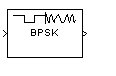# BPSK Modulator Baseband

Modulate using BPSK method

• Library:
• Communications Toolbox / Modulation / Digital Baseband Modulation / PM

Communications Toolbox HDL Support / Modulation / PM

•## Description

The BPSK Modulator Baseband block modulates a signal by using the binary phase shift keying (BPSK) method. The output is a baseband representation of the modulated signal. The input signal must be a discrete-time binary-valued signal. If the input bit is 0 or 1, then the modulated symbol is exp(jθ) or -exp(jθ), respectively. The ```Phase offset (rad)``` parameter specifies the value of θ in radians.

## Ports

### Input

expand all

Input signal, specified as a scalar or vector with element values in the range [0,M – 1], where M is the modulation order. If you specify a binary vector, the number of elements must be an integer multiple of the number of bits per symbol. The number of bits per symbol is equal to log2(M).

Data Types: `single` | `double` | `int8` | `int16` | `int32` | `uint8` | `uint16` | `uint32` | `Boolean`
Complex Number Support: Yes

### Output

expand all

BPSK-modulated baseband signal, returned as a complex-valued scalar or vector.

Data Types: `single` | `double` | `fixed point`

## Parameters

expand all

Main

Phase offset of the zeroth point of the constellation in radians, specified as a scalar.

Example: `pi/4`

Data Types

Output data type, specified as one of these options.

• `double`

• `single`

• `Inherit via back propagation` — The block matches the output data type and scaling to the next block in the model

• `fixdt(1,16)`

• `fixdt(1,16,0)`

• `<data type expression>` — Enables parameters for which you specify additional details

## Block Characteristics

 Data Types `Boolean` | `double` | `fixed pointa, b` | `integer` | `single` Multidimensional Signals `no` Variable-Size Signals `yes` a ufix(ceil(log2(M))) only at the input for M-ary modulation.b Fixed-point outputs must be signed.

expand all

## Algorithms

Phase modulation is a linear baseband modulation technique in which the message modulates the phase of a constant amplitude signal. Binary Phase Shift Keying (BPSK) is a two phase modulation scheme, where the 0’s and 1’s in a binary message are represented by two different phase states in the carrier signal

`${s}_{n}\left(t\right)=\sqrt{\frac{2{E}_{b}}{{T}_{b}}}\mathrm{cos}\left(2\pi {f}_{c}t+{\varphi }_{n}\right),$`

for where:

• ϕn = πm, m∈{0,1}.

• Eb is the energy per bit.

• Tb is the bit duration.

• fc is the carrier frequency.

In MATLAB®, the baseband representation of a BPSK signal is

`${s}_{n}\left(t\right)={e}^{-i{\varphi }_{n}}=\mathrm{cos}\left(\pi n\right).$`

The BPSK signal has two phases: 0 and π. The probability of a bit error in an AWGN channel is

`${P}_{b}=Q\left(\sqrt{\frac{2{E}_{b}}{{N}_{0}}}\right),$`

where N0 is the noise power spectral density.

## Version History

Introduced before R2006a